Introduction to Response Surface Experiments

Many industrial experiments are conducted to discover which values of given factor variables optimize a response. If each factor is measured at three or more values, a quadratic response surface can be estimated by least squares regression. The predicted optimal value can be found from the estimated surface if the surface is shaped like a simple hill or valley. If the estimated surface is more complicated, or if the predicted optimum is far from the region of experimentation, then the shape of the surface can be analyzed to indicate the directions in which new experiments should be performed.

Suppose that a response variable y is measured at combinations of values of two factor variables,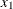and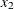. The quadratic response surface model for this variable is written as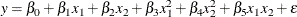The steps in the analysis for such data are as follows:

1. model fitting and analysis of variance, including lack-of-fit testing, to estimate parameters

2. canonical analysis to investigate the shape of the predicted response surface

3. ridge analysis to search for the region of optimum response

Model Fitting and Analysis of Variance

The first task in analyzing the response surface is to estimate the parameters of the model by least squares regression and to obtain information about the fit in the form of an analysis of variance. The estimated surface is typically curved: a hill with the peak occurring at the unique estimated point of maximum response, a valley, or a saddle surface with no unique minimum or maximum. Use the results of this phase of the analysis to answer the following questions:

• What is the contribution of each type of effect—linear, quadratic, and crossproduct—to the statistical fit? The ANOVA table with sources labeled Regression addresses this question.

• What part of the residual error is due to lack of fit? Does the quadratic response model adequately represent the true response surface? If you specify the LACKFIT option in the MODEL statement, then the ANOVA table with sources labeled Residual addresses this question. See the section Lack-of-Fit Test for details.

• What is the contribution of each factor variable to the statistical fit? Can the response be predicted accurately if the variable is removed? The ANOVA table with sources labeled Factor addresses this question.

• What are the predicted responses for a grid of factor values? (See the section Plotting the Surface and the section Searching for Multiple Response Conditions.)

Lack-of-Fit Test

The lack-of-fit test compares the variation around the model with pure variation within replicated observations. This measures the adequacy of the quadratic response surface model. In particular, if there are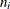replicated observations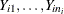of the response all at the same values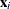of the factors, then you can predict the true response ateither by using the predicted value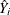based on the model or by using the mean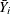of the replicated values. The lack-of-fit test decomposes the residual error into a component due to the variation of the replications around their mean value (the pure error) and a component due to the variation of the mean values around the model prediction (the bias error):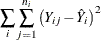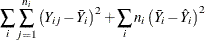If the model is adequate, then both components estimate the nominal level of error; however, if the bias component of error is much larger than the pure error, then this constitutes evidence that there is significant lack of fit.

If some observations in your design are replicated, you can test for lack of fit by specifying the LACKFIT option in the MODEL statement. Note that, since all other tests use total error rather than pure error, you might want to hand-calculate the tests with respect to pure error if the lack of fit is significant. On the other hand, significant lack of fit indicates that the quadratic model is inadequate, so if this is a problem you can also try to refine the model, possibly by using PROC GLM for general polynomial modeling; see Chapter 42: The GLM Procedure, for more information. Example 81.1 illustrates the use of the LACKFIT option.

Canonical Analysis

The second task in analyzing the response surface is to examine the overall shape of the curve and determine whether the estimated stationary point is a maximum, a minimum, or a saddle point. The canonical analysis can be used to answer the following questions:

• Is the surface shaped like a hill, a valley, or a saddle, or is it flat?

• If there is a unique optimum combination of factor values, where is it?

• To which factor or factors are the predicted responses most sensitive?

The eigenvalues and eigenvectors in the matrix of second-order parameters characterize the shape of the response surface. The eigenvectors point in the directions of principal orientation for the surface, and the signs and magnitudes of the associated eigenvalues give the shape of the surface in these directions. Positive eigenvalues indicate directions of upward curvature, and negative eigenvalues indicate directions of downward curvature. The larger an eigenvalue is in absolute value, the more pronounced is the curvature of the response surface in the associated direction. Often, all the coefficients of an eigenvector except for one are relatively small, indicating that the vector points roughly along the axis associated with the factor corresponding to the single large coefficient. In this case, the canonical analysis can be used to determine the relative sensitivity of the predicted response surface to variations in that factor. (See the section Getting Started: RSREG Procedure for an example.)

Ridge Analysis

If the estimated surface is found to have a simple optimum well within the range of experimentation, the analysis performed by the preceding two steps might be sufficient. In more complicated situations, further search for the region of optimum response is required. The method of ridge analysis computes the estimated ridge of optimum response for increasing radii from the center of the original design. The ridge analysis answers the following question:

• If there is not a unique optimum of the response surface within the range of experimentation, in which direction should further searching be done in order to locate the optimum?

You can use the RIDGE statement to compute the ridge of maximum or minimum response.